# Adding And Subtracting Surds Worksheet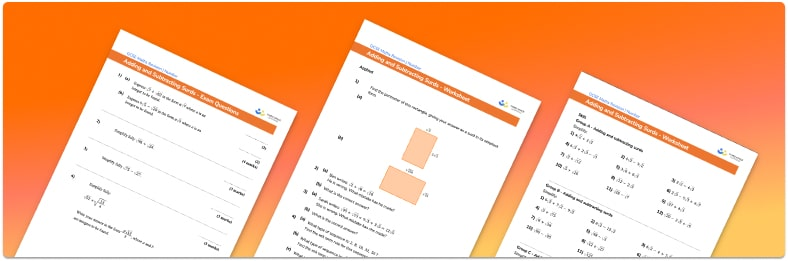• This field is for validation purposes and should be left unchanged.

You can unsubscribe at any time (each email we send will contain an easy way to unsubscribe). To find out more about how we use your data, see our privacy policy.

• Section 1 of the adding and subtracting surds worksheet contains 36 skills-based adding and subtracting surds questions, in 3 groups to support differentiation
• Section 2 contains 4 applied adding and subtracting surds questions with a mix of worded problems and deeper problem solving questions
• Section 3 contains 4 foundation and higher level GCSE exam style questions on adding and subtracting surds
• Answers and a mark scheme for all adding and subtracting surds questions are provided
• Questions follow variation theory with plenty of opportunities for students to work independently at their own level
• All questions created by fully qualified expert secondary maths teachers
• Suitable for GCSE maths revision for AQA, OCR and Edexcel exam boards

### Adding and subtracting surds at a glance

Surds are numbers left as square roots. They are used to give exact values for answers which are square roots of non square numbers. Surds are irrational numbers which means that, when written as decimals, they go on forever without any pattern. Irrational numbers cannot be written as fractions with integer numerators and denominators.

To add and subtract surds, the number under the root sign must be the same. We can only add or subtract two surds with different numbers under the roots if we can simplify one or both of them so that they have the same number under the root.

After we have added or subtracted the surds we can simplify surds by taking out factors which are square numbers. Surds are in their simplest form when the number under the root sign has no factors which are square numbers.

Looking forward, students can then progress to additional number worksheets, for example aor aFor more teaching and learning support on Number our GCSE maths lessons provide step by step support for all GCSE maths concepts.

## Related worksheets

Rational And Irrational Numbers Worksheet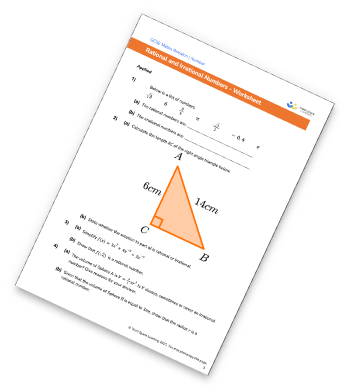Surds Worksheet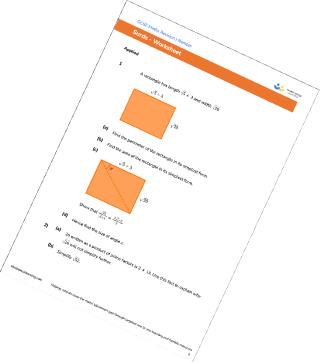Rationalise The Denominator Worksheet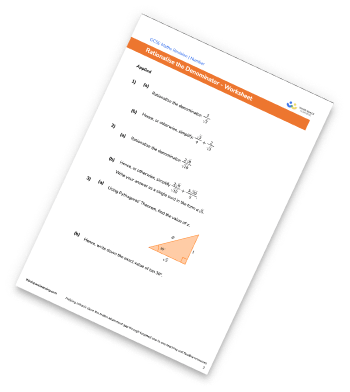Multiplying And Dividing Surds Worksheet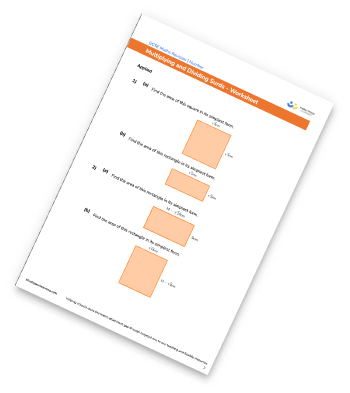## Do you have KS4 students who need more focused attention to succeed at GCSE?There will be students in your class who require individual attention to help them succeed in their maths GCSEs. In a class of 30, it’s not always easy to provide.

Help your students feel confident with exam-style questions and the strategies they’ll need to answer them correctly with our dedicated GCSE maths revision programme.

Lessons are selected to provide support where each student needs it most, and specially-trained GCSE maths tutors adapt the pitch and pace of each lesson. This ensures a personalised revision programme that raises grades and boosts confidence.

Find out more